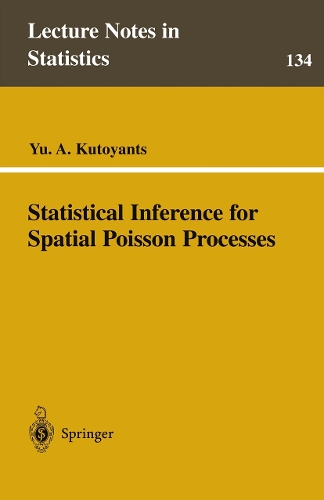•Statistical Inference for Spatial Poisson Processes - Lecture Notes in Statistics 134 (Paperback)

(author)
£109.99
Paperback 279 Pages / Published: 11/09/1998
• We can order this

Usually dispatched within 3 weeks

This work is devoted to several problems of parametric (mainly) and nonparametric estimation through the observation of Poisson processes defined on general spaces. Poisson processes are quite popular in applied research and therefore they attract the attention of many statisticians. There are a lot of good books on point processes and many of them contain chapters devoted to statistical inference for general and partic- ular models of processes. There are even chapters on statistical estimation problems for inhomogeneous Poisson processes in asymptotic statements. Nevertheless it seems that the asymptotic theory of estimation for nonlinear models of Poisson processes needs some development. Here nonlinear means the models of inhomogeneous Pois- son processes with intensity function nonlinearly depending on unknown parameters. In such situations the estimators usually cannot be written in exact form and are given as solutions of some equations. However the models can be quite fruitful in en- gineering problems and the existing computing algorithms are sufficiently powerful to calculate these estimators. Therefore the properties of estimators can be interesting too.

Publisher: Springer-Verlag New York Inc.
ISBN: 9780387985626
Number of pages: 279
Weight: 910 g
Dimensions: 235 x 155 x 15 mm
Edition: Softcover reprint of the original 1st ed. 199